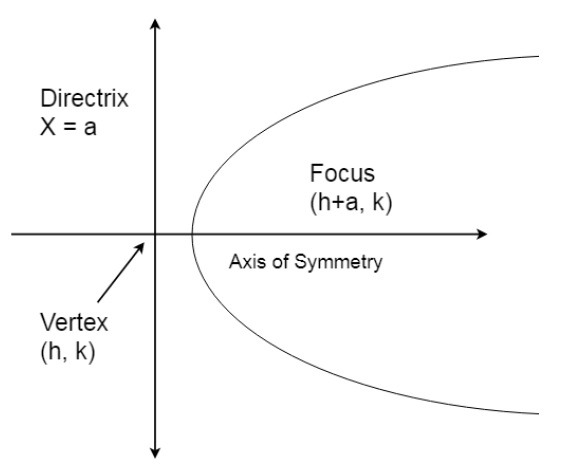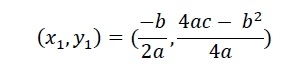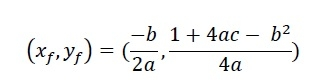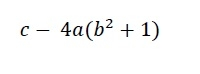# C/C++ Program for Finding the vertex, focus and directrix of a parabola?

CServer Side ProgrammingProgrammingC++

#### C in Depth: The Complete C Programming Guide for Beginners

45 Lectures 4.5 hours

#### Practical C++: Learn C++ Basics Step by Step

Most Popular

50 Lectures 4.5 hours

#### Master C and Embedded C Programming- Learn as you go

66 Lectures 5.5 hours

Here we will see how to find the vertex, focus directrix of a parabola using C or C++ program. To get these parameters we need the general equation of a parabola. The general formula is −

𝑦 = 𝑎𝑥2 + 𝑏𝑥 + 𝑐

The values of a, b and c are given.The formula for the vertex −The formula for the focus −The formula for the Directrix - y −## Example

Live Demo

#include <bits/stdc++.h>
using namespace std;
void getParabolaDetails(float a, float b, float c) {
cout << "The vertex: (" << (-b / (2 * a)) << ", " << (((4 * a * c) - (b * b)) / (4 * a)) << ")" << endl;
cout << "The Focus: (" << (-b / (2 * a)) << ", " << (((4 * a * c) - (b * b) + 1) / (4 * a)) << ")" << endl;
cout << "y-Directrix:" << c - ((b * b) + 1) * 4 * a << endl;
}
main() {
float a = 10, b = 3, c = 4;
}
The vertex: (-0.15, 3.775)
y-Directrix:-396